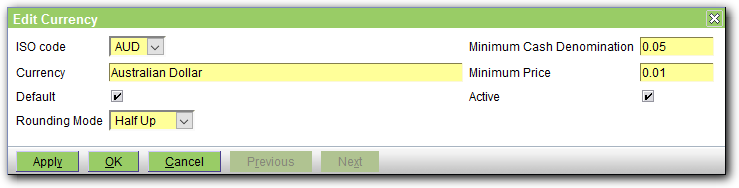# Currency

This screen is used to create/view/edit the details of the Currency lookups. Note that this is used in only one place, to set the currency used by the practice. The system runs in single currency mode and there is no support for foreign currency transactions.The fields are as follows:

ISO code - the code for the currency. When creating the Currency, the pull-down list allows you to select from the standard set (eg see http://en.wikipedia.org/wiki/ISO_4217). Note that once you have created the Currency Lookup, you cannot change the Code - you have to create a new one, and then use the Replace mechanism.
Currency - the name of the currency
Default - check the box to make this the default currency
Rounding Mode - this determines how amounts are rounded. It can be set to Half Down, Half Up or Half Even. See below for more.
Minimum Cash Denomination - the value of the smallest coin in this currency
Minimum Price - the minimum price to round to. This can be used to round prices to the nearest 5 cents or dollar for example. If unset or zero, all prices will be rounded to the default number of decimal places for the currency. (This is specified for each currency code - see the 3rd column (E) in the list of codes at https://en.wikipedia.org/wiki/ISO_4217#Active_codes which is 2 for most but 0 for some such as the Chilean Peso).
Active - uncheck the box to deactivate the currency

OpenVPMS assumes that all currencies are decimal and displays amounts to two decimal places, ie it assumes a dollars and cents structure. When doing calculations (say for a 20% discount on an amount of \$23.57) then commonly the calculation will not yield an exact number of cents (in this case the discount amount is 23.57/5=4.714). The Rounding Mode determines how the fractional cents are rounded as follows:

 Half Up Round to nearest cent, for half a cent, round up away from zero. Note that this is the rounding mode that most of us were taught at school. 4.714->4.71 4.715->4.72 4.716->4.72 -4.714->-4.71 -4.715->-4.72 -4.416->-4.72 Half Down Round to nearest cent, for half a cent, round down towards zero. 4.715->4.72 -4.715->-4.71 Half Even Round to nearest cent, for half a cent, round towards the even cent. Note that this is the rounding mode that minimizes cumulative error when applied repeatedly over a sequence of calculations. 4.715->4.72 4.725->4.72 -4.715->-4.72 -4.725->-4.72